# ICSE Solutions for Selina Concise Chapter 14 Rectilinear Figures Class 9 Maths

### Exercise 14(A)

1. The sum of the inteior angles of a polygon is four times the sum of its exterior angles. Find the number of sides in the polygon.

The sum of the interior angle=4 times the sum of the exterior angles.

Therefore the sum of the interior angles = 4×360° =1440°.

Now we have

(2n-4)×90° = 144°
⇒ 2n - 4= 16
⇒ 2n = 20
⇒ n = 10
Thus, the number of sides in the polygon is 10.

2. The angles of a pentagon are in the ratio 4 : 8 : 6 : 4 : 5. Find each angle of the pentagon.

Let the angles of the pentagon are 4x, 8x, 6x, 4x and 5x.

Thus we can write

4x+8x+6x+4x+5x = 540°
⇒ 27x = 540°
⇒ x = 20°
Hence the angles of the pentagon are :
4×20°= 80°, 8×20° = 160°, 6×20° = 120°, 4×20° = 80°, 5×20° = 100°

3. One angle of a six-sided polygon is 140o and the other angles are equal. Find the measure of each equal angle.

Let the measure of each equal angles are x.

Then we can write

140° + 5x = (2×6-4)×90°
⇒ 140° + 5x = 720°
⇒ 5x = 580°
⇒ x = 116°

Therefore, the measure of each equal angles are 116°.

4. In a polygon there are 5 right angles and the remaining angles are equal to 195o each. Find the number of sides in the polygon.

Let the number of sides of the polygon is n and there are k angles with measure 195o.

Therefore we can write:

5×90° + k×195° = (2n-4)90°
⇒ 180°n - 195°k = 450° - 360°
⇒ 180°n - 195°k = 90°
⇒ 12n - 13k = 6

In this linear equation n and k must be integer. Therefore to satisfy this equation the minimum value of k must be 6 to get n as integer.

Hence, the number of sides are: 5 + 6 = 11.

5. Three angles of a seven sided polygon are 132o each and the remaining four angles are equal. Find the value of each equal angle.

Let the measure of each equal angles are x.

Then we can write:

3×132° + 4x = (2×7-4)90°
⇒ 4x = 900°- 396°
⇒ 4x = 504
⇒ x =126°

Thus, the measure of each equal angles are 126°.

6. Two angles of an eight sided polygon are 142o and 176o. If the remaining angles are equal to each other; find the magnitude of each of the equal angles.

Let the measure of each equal sides of the polygon is x.

Then we can write:

142°+176°+6x = (2×8-4)90°
⇒ 6x = 1080°- 318°
⇒ 6x = 762°
⇒ x = 127°

Thus, the measure of each equal angles are 127o.

7. In a pentagon ABCDE, AB is parallel to DC and ∠A : ∠E : ∠D = 3 : 4 : 5. Find angle E.

Let the measure of the angles are 3x, 4x and 5x.

Thus

∠A + ∠B + ∠C +∠D + ∠E = 540°
⇒ 3x +(∠B+∠C) + 4x+ 5x = 540°
⇒ 12x + 180° = 540°
⇒ 12x = 360°
⇒ x = 30°
Thus, the measure of angle E will be 4×30°=120°

8. AB, BC and CD are the three consecutive sides of a regular polygon. If BAC = 15o; find,

(i) Each interior angle of the polygon.

(ii) Each exterior angle of the polygon.

(iii) Number of sides of the polygon

(i) Let each angle of measure x degree.

Therefore, measure of each angle will be:

x =180° - 2×15° = 150°

(ii) Let each angle of measure x degree.
Therefore measure of each exterior angle will be
x = 180° - 150° = 30°

(iii) Let the number of each sides is n.
Now we can write,
n×150° = (2n -4) ×90°
⇒ 180°n -150°n = 360°
⇒ 30°n = 360°
⇒ n = 12

Thus, the number of sides are 12.

9. The ratio between an exterior angle and an interior angle of a regular polygon is 2 : 3. Find the number of sides in the polygon.

Let measure of each interior and exterior angles are 3k and 2k.

Let number of sides of the polygon is n.

Now we can write:

n×3k = (2n-4)×90°
⇒ 3nk = (2n - 4)90°  ...(1)
Again,
n×2k = 360°
⇒ nk = 180°
From(1)
3×180° = (2n - 4)90°
⇒ 3 = n-2
⇒ n = 5

Thus the number of sides of the polygon is 5.

10. The difference between an exterior angle of (n – 1) sided regular polygon and an exterior angle of (n + 2) sided regular polygon is 6o find the value of n.

For (n-1) sided regular polygon:

Let measure of each angle is x.

Therefore

(n-1)x = [2(n-1)-4]90°

Thus, the value of n is 13.

11. Two alternate sides of a regular polygon, when produced, meet at right angle. Find:

(i) The value of each exterior angle of the polygon;

(ii) The number of sides in the polygon.

(i) Let the measure of each exterior angle is x and the number of sides is n.

Therefore we can write:
n = 360°/x

Now we have,
x+x+90° = 180°
⇒ 2x = 90°
⇒ x =45°

(ii) Thus the number of sides in the polygon is

n = 360°/45° = 8°
Required number of side of regular polygon = 8

### Exercise 14(B)

1. State, ‘true’ or ‘false’

(i) The diagonals of a rectangle bisect each other.

(ii) The diagonals of a quadrilateral bisect each other.

(iii) The diagonals of a parallelogram bisect each other at right angle.

(iv) Each diagonal of a rhombus bisects it.

(v) The quadrilateral, whose four sides are equal, is a square.

(vi) Every rhombus is a parallelogram.

(vii) Every parallelogram is a rhombus.

(viii) Diagonals of a rhombus are equal.

(ix) If two adjacent sides of a parallelogram are equal, it is a rhombus.

(x) If the diagonals of a quadrilateral bisect each other at right angle, the quadrilateral is a square.

(i) True.

This is true, because we know that a rectangle is a parallelogram. So, all the properties of a parallelogram are true for a rectangle. Since the diagonals of a parallelogram bisect each other, the same holds true for a rectangle.

(ii) False

This is not true for any random quadrilateral. Observe the quadrilateral shown below.

Clearly the diagonals of the given quadrilateral do not bisect each other. However, if the quadrilateral was a special quadrilateral like a parallelogram, this would hold true.

(iii) False

Consider a rectangle as shown below.

It is a parallelogram. However, the diagonals of a rectangle do not intersect at right angles, even though they bisect each other.

(iv) True

Since a rhombus is a parallelogram, and we know that the diagonals of a parallelogram bisect each other, hence the diagonals of a rhombus too, bisect other.

(v) False

This need not be true, since if the angles of the quadrilateral are not right angles, the quadrilateral would be a rhombus rather than a square.

(vi) True

A parallelogram is a quadrilateral with opposite sides parallel and equal.

Since opposite sides of a rhombus are parallel, and all the sides of the rhombus are equal, a rhombus is a parallelogram.

(vii) False

This is false, since a parallelogram in general does not have all its sides equal. Only opposite sides of a parallelogram are equal. However, a rhombus has all its sides equal. So, every parallelogram cannot be a rhombus, except those parallelograms that have all equal sides.

(viii) False

This is a property of a rhombus. The diagonals of a rhombus need not be equal.

(ix) True

A parallelogram is a quadrilateral with opposite sides parallel and equal.

A rhombus is a quadrilateral with opposite sides parallel, and all sides equal.

If in a parallelogram the adjacent sides are equal, it means all the sides of the parallelogram are equal, thus forming a rhombus.

(x) False

Observe the above figure. The diagonals of the quadrilateral shown above bisect each other at right angles, however the quadrilateral need not be a square, since the angles of the quadrilateral are clearly not right angles.

2. In the figure, given below, AM bisects angle A and DM bisects angle D of parallelogram ABCD. Prove that : AMD = 90o.

From the given figure we conclude that

∠A + ∠D = 180° [since consecutive angles are supplementary]
⇒ ∠A/2 + ∠D/2 = 90°
∠A/2 + ∠D/2 + ∠M = 180°
⇒ 90° +∠M = 180° [∴∠A/2 + ∠D/2 = 90°]
⇒ ∠M = 90°
Hence, ∠AMD = 90°

3. In the following figure, AE and BC are equal and parallel and the three sides AB, CD and DE are equal to one another. If angle A is 102o. Find angles AEC and BCD.

In the given figure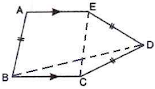Given that AE = BC
We have to find ∠AEC and ∠BCD
Let us join EC and BD.
AE = BC and AB = EC
Also, AE॥BC
⇒ AB॥ EC
In parallelogram consecutive angles are supplementary
⇒ ∠A + ∠B = 180°
⇒ 102° + ∠B = 180°
⇒ ∠B = 78°
In parallelogram opposite angles are equal
⇒ ∠A = ∠BEC and ∠B = ∠AEC
⇒ ∠BEC = 102° and ∠AEC = 78°
Now consider △ECD
EC = ED = CD  [Since AB = EC]
Therefore △ECD is an equilateral triangle.
⇒ ∠ECD = 60°
∠BCD = ∠BEC + ∠ECD
⇒ ∠BCD = 102° + 60°
⇒ ∠BCD = 162°
Therefore, ∠AEC = 78° and ∠BCD = 162°

4. In a square ABCD, diagonals meet at O. P is a point on BC such that OB = BP.

Show that:

(i) POC = 22(1/2)°

(ii) BDC = 2 POC

(iii) BOP = 3 CPO

Let ∠POC = x°
Diagonals of a square bisect the angles.
∴ ∠OCP = ∠OBP= 90°/2 = 45°
Using exterior angle property for △OPC,
∠OPB = ∠OCP + ∠POC
⇒ ∠OPB = 45° + x°  ...(i)
In △OBP,
OB = BP(given)
∴∠OPB = ∠BOP  (angles opposite to equal sides are equal)
⇒ ∠BOP = 45° + x° ...(ii) [From (i)]
Diagonals of a square are perpendicular to each other.
∴ ∠BOP + ∠POC = 90°
⇒ 45° + x° + x° = 90°
⇒ x = 22.5°
⇒ ∠POC = x° = 22(1/2)°
Now, ∠BDC = 45° (Diagonals of a square bisect the angles)
∠BDC = 2°×22.5° = 2∠POC
And, ∠BOP = 45° +x° [From (ii)]
∠BOP = 45°+ 22.5° = 3×22.5° = 3∠POC

5. The given figure shows a square ABCD and an equilateral triangle ABP. Calculate:

(i) ∠AOB

(ii) ∠BPC

(iii) ∠PCD

(iv) Reflex ∠APCIn the given figure △APB is an equilateral triangle.
Therefore all its angles are 60°.
⇒ ∠ABD = 45°
⇒ ∠AOB = 180°- 60° - 45° = 75°
Again in △BPC,
⇒ ∠BPC = 75° [Since BP = CB]
Now,
∠C = ∠BCP + ∠PCD
⇒ ∠PCD = 90° - 75°
⇒ ∠PCD = 15°
Therefore,
∠APC = 60° + 75°
⇒ ∠APC = 135°
⇒ Reflex ∠APD = 360° - 135° = 225°

6. In the given figure ABCD is a rhombus with angle A = 67o

If DEC is an equilateral triangle, calculate:

(i) ∠CBE

(ii) ∠DBE.

Given that the figure ABCD is a rhombus with angle A = 67o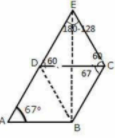Consider △DBC,
DC = CB  [Sides of rhombus]
So △DBC is an isosceles triangle
⇒ ∠CDB = ∠CBD
Also,
∠CDB + ∠CDB +∠BCD = 180°
⇒ 2∠CBD = 113°
⇒ ∠CDB = ∠CBD = 56.5° ...(i)
Consider △DCE,
EC = CB
So △DCE is an isosceles triangle
⇒ ∠CBE = ∠CEB
Also,
∠CBE + ∠CEB +∠BCE = 180°
⇒ 2∠CBE = 53°
⇒ ∠CDE = 26.5°
From (i)
∠CBD = 56.5°
⇒ ∠CBE + ∠DBE = 56.5°
⇒ 26.5° +∠DBE = 56.5°
⇒ ∠DBE = 30.5°

7. In each of the following figures, ABCD is a parallelogram.

(i)

(ii)

In each case, given above, find the values of x and y.

(i) ABCD is a parallelogram

Therefore

AB =DC
Thus
4y = 3x - 3  [since AD = BC]
⇒ 3x - 4y = 3 ...(i)
6y+2 =4x  [since AB =DC]
⇒ 4x - 6y = 2  ...(ii)
Solving equations(i) and (ii) we have
x = 5
y= 3

(ii) In the figure ABCD is a parallelogram
∠A = ∠C
∠B = ∠D  [since opposite angles are equal]
Therefore
7y = 6y+ 3y -8° ...(i) [Since ∠A =∠C]
⇒ 4x+20° = 0 ...(ii)
Solving (i), (ii) we have
x = 12°
y = 16°

8. The angles of a quadrilateral are in the ratio 3 : 4 : 5 : 6. Show that the quadrilateral is a trapezium.

Given that the angles of a quadrilateral are in the ratio Let the angles be
3x+4x+5x+ 6x = 360°
⇒ x = 360°/18
⇒ x = 20°
Therefore the angles are
3×20 = 60°
4×20 = 80°
5×20 = 100°
6×20 = 120°
Since all the angles are of different degrees thus forms a trapezium.

9. In a parallelogram ABCD, AB = 20 cm and AD = 12 cm. The bisector of angle A meets DC at E and BC produced at F. Find the length of CF.

Given, AB = 20 cm and AD = 12 cm.

From the above figure, it’s evident that ABF is an isosceles triangle with angle BAF = angle BFA = x

So AB = BF = 20

BF = 20

⇒ BC + CF = 20

⇒ CF = 20 – 12 = 8 cm

10. In parallelogram ABCD, AP and AQ are perpendiculars from vertex of obtuse angle A as shown. If angles x : y = 2:1; find angles of the parallelogram.

We know that AQCP is a quadrilateral. So sum of all angles must be 360.

∴ x + y + 90 + 90 = 360

⇒ x + y = 180

Given x:y = 2:1

So substitute x = 2y

3y = 180

⇒ y = 60

x = 120

We know that angle C = angle A = x = 120

Angle D = Angle B = 180 – x = 180 – 120 = 60

Hence, angles of parallelogram are 120, 60, 120 and 60.

### Exercise 14(C)

1. E is the mid-point of side AB and F is the mid point of side DC of parallelogram ABCD. Prove that AEFD is a parallelogram.

Let us draw a parallelogram ABCD where F is the midpoint of side DC of parallelogram ABCD
To prove : AEFD is a parallelogram

Therefore, AEFC is a parallelogram.

2. The diagonal BD of a parallelogram ABCD bisects angles B and D. Prove that ABCD is a rhombus.

Given: ABCD is a parallelogram where the diagonal BD bisects
parallelogram ABCD at angle B and D
To prove: ABCD is a rhombus
Proof: Let us draw a parallelogram ABCD where the diagonal BD bisects the parallelogram at angle B and D.
Construction: Let us join AC as a diagonal of the parallelogram ABCD

AB = DC
Diagonal BD bisects angle B and D
So, ∠COD = ∠DOA
Again,
AC also bisects at A and C
Therefore, ∠AOB = ∠BOC
Thus, ABCD is a rhombus.
Hence, proved.

3. The alongside figure shows a parallelogram ABCD in which AE = EF = FC. Prove that:

(i) DE is parallel to FB

(ii) DE = FB

(iii) DEBF is a parallelogram.

Construction:
Join DF and EB.
Join diagonal BD.Since, diagonals of a parallelogram bisect each other.
∴ OA = OC and OB = OD
Also, AE = EF = FC
Now, OA = OC and AE = FC
⇒ OA - AE = OC - FC
⇒ OE = OF
Thus, in quadrilateral DEFB, we have
OB = OD and OE = OF
⇒ Diagonals of a quadrilateral DEFB bisects each other.
⇒ DEFB is a parallelogram.
⇒ DE is parallel to FB
⇒ DE = FB  (Opposite sides are equal)

4. In the alongside diagram, ABCD is a parallelogram in which AP bisects angle AP bisects angle A and BQ bisects angle B. Prove that :

(i) AQ = BP

(ii) PQ = CD.

(iii) ABPQ is a parallelogram.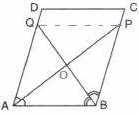Let us join PQ.
Consider the ΔAOQ and ΔBOP
∠AOQ = ∠BOP  [opposite angles]
∠OAQ = ∠BPO  [alternate angles]
⇒ ΔAOQ ≅ ΔBOP  [AA test]
Hence AQ  = BP
Consider the ΔQOP and ΔAOB
∠AOB = ∠QOP  [opposite angles]
∠OAB = ∠APQ  [alternate angles]
⇒ ΔQOP ≅ ΔAOB  [AA test]
Hence, PQ = AB = CD
DQ = CP and DQ॥ CP   [Since AD = BC and AD॥ BC]
Also,
QP = DC and AB॥QP॥DC
Hence, quadrilateral QPCD is a parallelogram.

5. In the given figure, ABCD is a parallelogram. Prove that: AB = 2 BC.

Given ABCD is a parallelogram
To prove AB = 2BC

Proof : ABCD is a parallelogram
∠A + ∠D  = ∠B + ∠C = 180
From the △AEB we have
∠A/2 + ∠B/2 + ∠E = 180
⇒ ∠A - ∠A/2 + ∠D + ∠E1 = 180  [taking E1 as new angle]
⇒ ∠A + ∠D + ∠E1 = 180 + ∠A/2
⇒ ∠E1 = ∠A/2  [Since ∠A + ∠D = 180]
Again similarly,
∠E2 = ∠B/2
Now
AB = DE + EC
= 2BC  [since AD = BC]
Hence, proved.

6. Prove that the bisectors of opposite angles of a parallelogram are parallel.

Given ABCD is a parallelogram. The bisectors of ∠ADC and ∠BCD meet at E. The bisectors of ∠ABC and ∠BCD meet at F.
From the parallelogram ABCD we have∠ADC + ∠BCD = 180°  [sum of adjacent angles of a parallelogram]
⇒ ∠ADC/2 + ∠BCD/2 = 90°
⇒ ∠EDC + ∠ECD = 90°
In triangle ECD sum of angles = 180°
∠EDC + ∠ECD + ∠CED = 180°
⇒ ∠CED = 90°
Similarly, taking triangle BCF it can be prove that ∠BFC = 90°
Now since,
∠BFC = ∠CED = 90°
Therefore, the lines DE and BF are parallel
Hence, proved.

7. Prove that the bisectors of interior angles of a parallelogram form a rectangle.

Given: ABCD is a parallelogram
BF bisects ∠ABC
CG bisects ∠BCD
To Prove: LKJI is a rectangle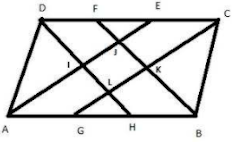Proof:
∠BAD + ∠ABC = 180°  [adjacent angles of a parallelogram are supplementary]
∠ABJ = (1/2)∠ABC [DH bisect ĐABC]
∠BAJ + ∠ABJ = 90°   [halves of supplementary angles are complementary]
△ABJ is a right triangle because its acute interior angles are complementary.
Similarly
∠DLC = 90°
∠AID = 90°
Then ∠JIL= 90 because ∠AID and ∠JIL are vertical angles
Since, 3 angles of quadrilateral LKJI are right angles, is the 4th one and so LKJI is a rectangle, since its interior angles are all right angles.

8. Prove that the bisectors of the interior angles of a rectangle form a square.

Given: A parallelogram ABCD in which AR,BR, CP, DP
Are the bisects of ∠A, ∠B, ∠C, ∠D respectively forming quadrilaterals PQRS.
To prove : PQRS is a rectangle

Proof:
∠DCB + ∠ABC = 180° [co-interior angles of parallelogram are supplementary]
⇒ (1/2)∠DCB + (1/2)∠ABC = 90°
⇒ ∠1 + ∠2 = 90°
△CQB, ∠1 + ∠2 + ∠CQB = 180°
From the above equation we get
∠CQB = 180° - 90° = 90°
∠RQP = 90° [∠CQB = ∠RQP, vertically opposite angles]
∠QRP = ∠RSP = ∠SPQ = 90°
Hence, PQRS is a rectangle

9. In parallelogram ABCD, the bisector of angle A meets DC at P and AB = 2 AD.

Prove that:

(i) BP bisects angle B.

(ii) Angle APB = 90o.

Also AP is the bisector ∠A
∠1 = ∠2
Now,
∠2 = ∠5  [alternate angles]
Therefore, ∠1 = ∠5
Now,
AP = DP = x  [sides opposite to equal angles are also equal]
Therefore,
AB = CD  [opposite sides of parallelogram are equal ]
CD = 2x
⇒ DP + PC = 2x
⇒ x + PC = 2x
⇒ PC = x
Also, BC = x
△BPC
⇒ ∠6 = ∠4 [angles opposite to equal sides are equal]
In ⇒ ∠6 = ∠3
Therefore, ∠3 = ∠4
Hence BP bisect ∠B
(ii) Opposite angles are supplementary
Therefore ,
∠1+ ∠2+∠3+∠4 = 180°
⇒ 2∠2 + 2∠3 = 180°  [∠1 = ∠2 , ∠3 = ∠4]
⇒ ∠2 + ∠3 = 90°
In △APB,
∠2 + ∠3+ ∠APB = 180°
⇒ ∠APB = 180° - 90°  [by angle sum property]
⇒ ∠APB = 90°
Hence, proved

10. Points M and N are taken on the diagonal AC of a parallelogram ABCD such that AM = CN. Prove that BMDN is a parallelogram.

Points M and N are taken on the diagonal AC of a parallelogram ABCD such that AM = CN.
Prove that BMDN is a parallelogram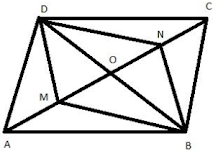Construction : Join B to D to meet AC in O.
Proof : we know that the diagonals of parallelogram bisect each other.
Now, AC and BD bisect each other at O.
OC = OA
AM = CN
⇒ OA - AM= OC - CN
⇒ OM = ON
Thus in a quadrilateral BMDN, diagonal BD and MN are such that OM = ON and OD = OB
Therefore, the diagonals AC and PQ bisect each other.
Hence, BMDN is a parallelogram.

11. In the following figure, ABCD is a parallelogram. Prove that:

(i) AP bisects angle A

(ii) BP bisects angle B

(iii) Angle DAP + Angle CBP = Angle APBAD = BC  [since ABCD is a parallelogram]
DC = AB  [since ABCD is a parallelogram]
∠A = ∠C  [opposite angles]
Therefore AP = BP
AP bisects ∠A
BP bisects ∠B
In △APB,
AP = PB
∠APB = ∠DAP + ∠BCP
Hence, proved

12. ABCD is a square. A is joined to a point P on BC and D is joined to a point Q on AB. If AP = DQ; prove that AP and DQ are perpendicular to each other.

ABCD is a square and AP = PQ
Consider △DAQ and △ABP
∠DAQ = ∠ABP = 90°
DQ = AP
△DAQ ≅ △ABP
⇒ ∠PAB = ∠QDA
Now,
∠PAB + ∠APB = 90°
Also, ∠QDA + ∠APB = 90°  [∠PAB = ∠QDA]
Consider △AOQ By ASP
∠QDA + ∠APB + ∠AOD = 180°
⇒ 90° + ∠AOD = 180°
∠AOD = 90°
Hence, AP and DQ are perpendicular.

13. In a quadrilateral ABCD, AB = AD and CB = CD. Prove that :

(ii) AC is perpendicular bisector of BD.

CB = CD
To prove: (i) AC bisects angle BAD.
(ii) AC is perpendicular bisector of BD.
Proof:

CB = CD  [given]
AC = AC  [common side]
OD = OB
OA  = OA [diagonals bisects each other at O]
Thus, AC is perpendicular bisector of BD
Hence, proved

14. The following figure shows a trapezium ABCD in which AB is parallel to DC and AD = BC.
Prove that:

(i) ∠DAB = ∠CBA

(iii) AC = BD

(iv) OA = OB and OC = OD

Given: ABCD is a trapezium, AB॥ DC and AD = BC
To prove:
(i) ∠DAB = ∠CBA
(iii) AC = BD
(iv) OA = OB and OC = OD
Proof:

(i) Since AD॥ CE and transversal AE cuts them at A and E respectively.
Therefore, ∠A + ∠B = 180°
Since, AB॥ CD and AD॥ BC
Therefore, ABCD is a parallelogram
∠A = ∠C
∠B = ∠D  [since ABCD is a parallelogram]
Therefore ∠DAB = ∠CBA
In △ABC and △BAD, we have
AB =BA   [common]
∠A = ∠B  [proved]
Therefore, AC = BD [corresponding parts of congruent triangles are equal]
OA = OB
Again, OC = OD [since diagonals bisect each other at O]
Hence, proved.

15. In the given figure, AP is bisector of ∠A and CQ is bisector of ∠C of parallelogram ABCD. Prove that APCQ is a parallelogram.

Construction: Join AC.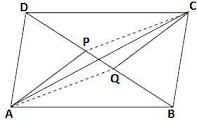Proof :
∠BAP = (1/2)∠A  (AP is the bisector of ∠A)
∠DCQ = (1/2)∠C  (CQ is the bisector of ∠C)
⇒ ∠BAP = ∠DCQ  ...(i) [∠A = ∠R (Opposite angles of a parallelogram)]
Now, ∠BAC = ∠DCA  ...(ii) [Alternate angles since AB॥DC]
Subtracting (ii) from (i), we get
∠BAP - ∠BAC = ∠DCQ - ∠DCA
⇒ ∠CAP = ∠ACQ
⇒ AP॥ QC  (Alternate angles are equal)
Similarly, PC॥ AQ
Hence, APCQ is a parallelogram.

16. In case of a parallelogram prove that:

(i) The bisectors of any two adjacent angles intersect at 90o.

(ii) The bisectors of opposite angles are parallel to each other.ABCD is a parallelogram, the bisectors of ∠ADC and
∠BCD meet at a point E and the bisectors of ∠BCD and ∠ABC meet at F.
We have to prove that the ∠CED = 90° and ∠CFG = 90°
Proof : In the parallelogram ABCD
∠ADC + ∠BCD = 180°  [sum of adjacent angles of a parallelogram]
⇒ ∠ADC/2 + ∠BCD/2 = 90°
⇒ ∠EDC + ∠ECD + ∠CED = 180°
⇒ ∠CED = 90°
Similarly, taking triangle BCF it can be proved that ∠BFC  = 90°
∠BFC + ∠CFG = 180°  [adjacent angles on a line]
Also, ∠CFG = 90°
Now, since ∠CFG = ∠CED = 90° [it means that the lines DE and BG are parallel]
Hence, proved.

17. The diagonals of a rectangle intersect each other at right angles. Prove that the rectangle is a square.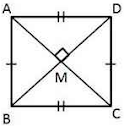To prove: ABCD is a square, that is, to prove that sides of the quadrilateral are equal and each angle of the quadrilateral is 90°,
ABCD is a rectangle,
∠A = ∠B = ∠C = ∠D = 90° and diagonals bisects each other that is , MD = BM   ...(i)
Consider △AMD and △AMB,
MD = BM  [from (i)]
∠AMD = ∠AMB = 90° (given)
AM = AM  (common side)
△AMD ≅ △AMB  (SAS congruence criterion)
Since, ABCD is a rectangle, AD = BC and AB = CD
Thus, AB = BC = CD = AD and ∠A = ∠B = ∠C = ∠D = 90°
⇒ ABCD is a square.

18. In the following figure, ABCD and PQRS are two parallelograms such that ∠D = 120° and ∠Q = 70°. Find the value of x.

ABCD is a parallelogram
⇒ opposite angles of a parallelogram are congruent
⇒ ∠DAB = ∠BCD and ∠ABC = ∠ADC = 120°
In ABCD,
∠DAB + ∠BCD + ∠ABC+ ∠ADC=  360° (sum of the measures of angles of a quadrilateral)
⇒ ∠BCD + ∠BCD + 120 + 120 = 360°
⇒ 2∠BCD = 360° - 240°
⇒ 2∠BCD = 120°
⇒ ∠BCD = 60°
PQRS is a parallelogram
⇒ ∠PQR = ∠PSR = 70°
In △CMS,
∠CMS + ∠CSM + ∠MCS = 180° (angle sum property)
⇒ x + 70° + 60° = 180°
⇒ x = 50°

19. In the following figure, ABCD is a rhombus and DCFE is a square.

If ∠ABC =56°, find:

(i) ∠DAE

(ii) ∠FEA

(iii) ∠EAC

(iv) ∠AEC

ABCD is a rhombus.

DCFE is a square.
⇒ ED = CD and ∠FED = ∠EDC= ∠DCF = ∠CFE = 90°

⇒ ∠DAE = ∠AED  ...(i)
∠DAE + ∠AED + ∠ADE = 180°
⇒ 2∠DAE + 146° = 180° (Since ∠ADE = ∠EDC + ∠ADC = 90° + 56° = 146°)
⇒ 2∠DAE = 34°
⇒ ∠DAE = 17°
⇒ ∠DEA = 17° ...(ii)
In ABCD,
∠ABC + ∠BCD + ∠ADC + ∠DAB = 360°
⇒ 56° + 56°+ 2∠DAB = 360°  (∵ opposite angles of rhombus are equal)
⇒ 2∠DAB = 248°
⇒ ∠DAB = 124°
We know that diagonals of a rhombus, bisect its angles.
∠DAC = 124°/2 = 62°
⇒ ∠EAC = ∠DAC - ∠DAE = 62° - 17° = 45°
Now, ∠FEA = ∠FED - ∠DEA
= 90° - 17°  [from (ii) and each angle of a square is 90°]
= 73°
We know that diagonals of a square bisect its angles.
∠CED = 90°/2 = 45°
So, ∠AEC = ∠CED - ∠DEA
= 45° - 17°
= 28°
Hence, ∠DAE = 17°, ∠FEA = 73°, ∠EAC = 45° and ∠AEC = 28°.